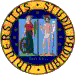#Prof. Yves André (Institut de Math. de Jussieu)
Volumes, complexity, transcendence

March 31, 2015

Abstract

Many mathematical constants can be expressed as integrals of rational functions with rational coefficients, possibly multiplied by an exponential, over domains defined by polynomial inequalities with rational coefficientsl. In the absence of exponential, such numbers can be expressed as volumes of solids defined by polynomial inequalities with rational coefficients. We shall consider their arithmetic properties from the double viewpoint of complexity theory and of transcendental number theory. Ultimately, they are related to deep questions concerning motives in atrithmetical geometry.

Short bio

Born in 1959. Ph.D. in 1984 at the University of Paris VI under the supervisor of Daniel Bertrand. Thesis title: Structure de Hodge, Differential Equations p-adiques, et indépendance algébrique de périodes d'intégrales abéliennes.
Directeur de recherche, Institut de Math. de Jussieu.
He works in several areas of Algebraic Geometry and Number Theory, from the theory of differential equations to the theory of motives.
He is the author of several research papers and outstanding books, among which: - Une introduction aux motifs, Société Mathématique de France, 2004. - De Rham cohomology of differential modules on algebraic varieties (with F. Baldassarri) Progress in Mathematics, 189, 2001.
He proved several important conjectures in algebraic geometry and number theory: - A conjecture of Crew on almost unipotence connection modules with Frobenius structure on the ring Robba; - A conjecture of Dwork on the logarithmic growth of solutions of p-adic differential equations; - A Conjecture of Malgrange on the variation of the irregularity in analytic family of linear differential equations with singularities méromorphes.
With Frans Oort he is the author of the famous André-Oort conjecture on special subvarieties of Shimura varieties.
In 2011 he was awarded the Prix Paul-Émile Doistau by the Academie des Sciences (see http://www.cnrs.fr/insmi/spip.php?article328).